Mixed Cell References in MS Excel

• Last Updated : 29 Sep, 2021

Cell: The row and column in Excel together make a cell. We input the value/data(s) in cells of a spreadsheet. There are three types of values that can be entered into the cell.

• Numbers
• Alphabetic
• Formulas

Cell reference ​​​​​​is the address or name of a cell or a range of cell is known as Cell reference. It is the combination of column name and row number. It helps the software to identify the cell from where the data/value is to be used in the formula. We can reference the cell of other worksheets and also of other programs.

• Referencing the cell of other worksheets is known as External referencing.
• Referencing the cell of other programs is known as Remote referencing.

There are two types of cell references in Excel:

1. Relative reference.
2. Absolute reference.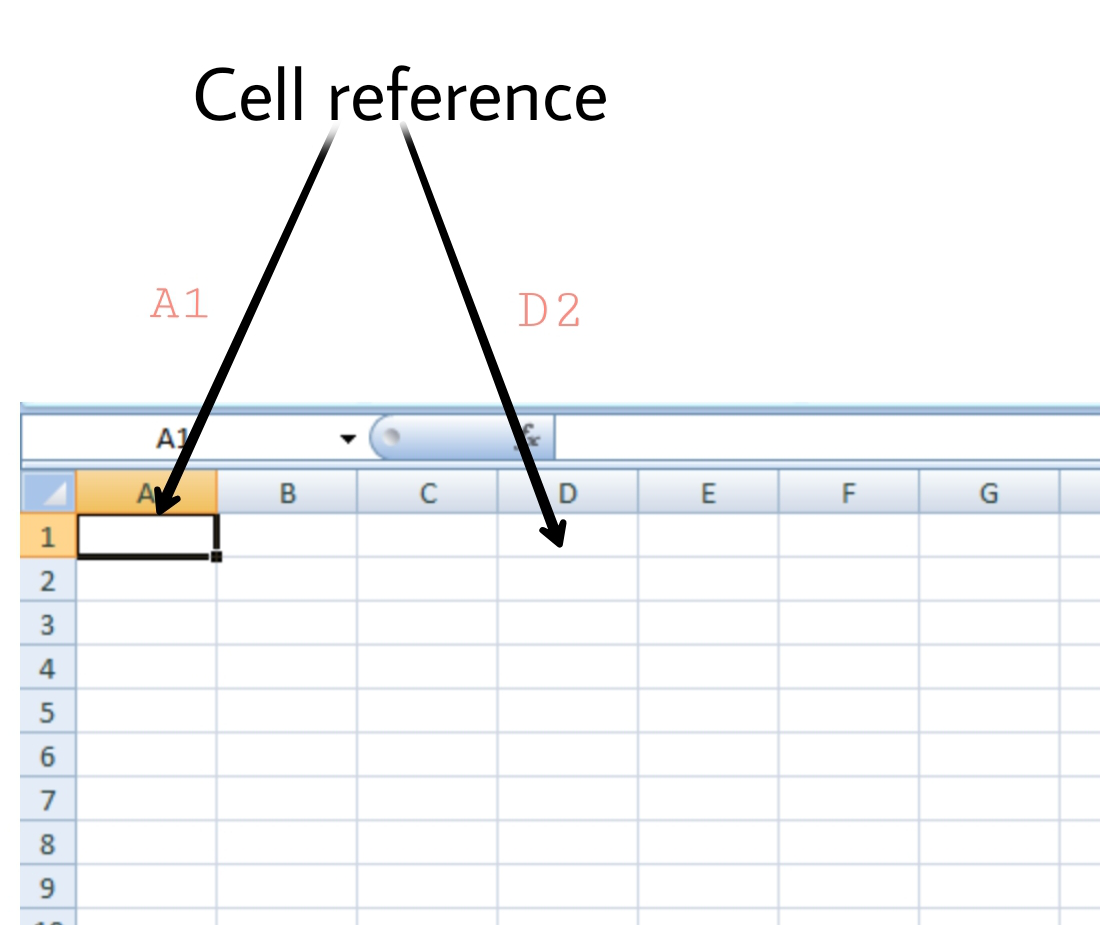Mixed Reference

Mixed Reference is a type of Absolute reference in which either the column is made constant or the row is made constant. When we make any column or row constant then the column name or row number does not change as we copy the formula to other cell(s). The mixed reference is designated by a dollar sign(\$) in front of the row or column. For example:

• \$F1: In this the column F is constant.
• F\$1: In this the row 1 is constant.

In another example say, we want to add the data of cell A2 with the cell B2 and B3 and display sum in C2 and C3 respectively. We do not make any row or column constant.

Steps to perform Mixed Reference:

Step 1: Write the formula in any cell. We write the formula(=B2+A2) in cell C2 and press enter.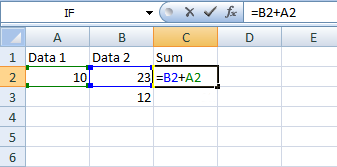Step 2: Click and hold on to the Fill handle.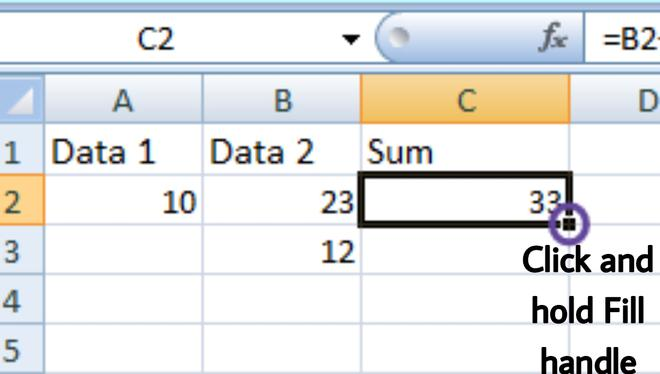Step 3: Drag it to the cell you want to fill. We drag it till cell C3.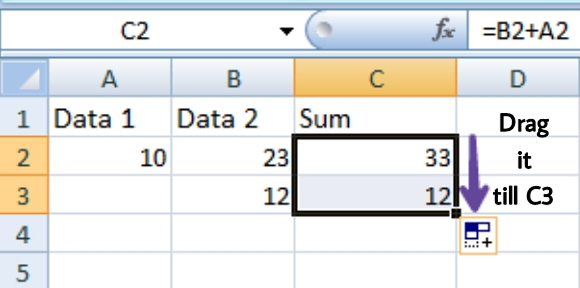Step 4: We did not make row 2 of column A constant, thus we see that as we copy the formula to cell C3, A2 changes to A3 i.e. the addition is performed between A2 and B2, A3 and B3.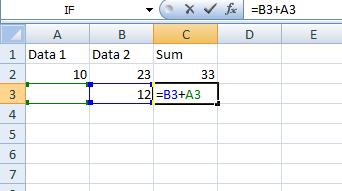We make row 2 of column A constant by adding a dollar sign before 2.

Step 5: Write the formula in any cell. We write the formula(=B2+A\$2) in cell C2 and press enter.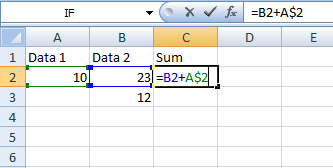Step 6: Click and hold on to the Fill handle.Step 7: Drag it to the cell you want to fill. We drag it till cell C3.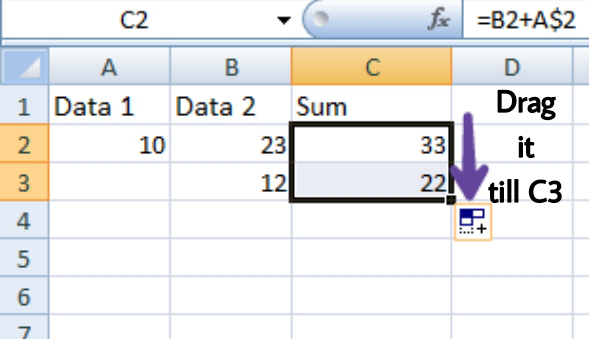Step 8: We made row 2 of column A constant, thus we see that as we copy the formula to cell C3, A2 does not change to A3 i.e. A2 is added to cell B2 and B3.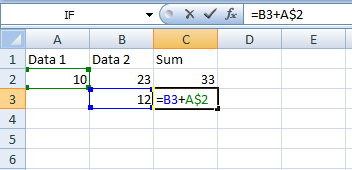My Personal Notes arrow_drop_up